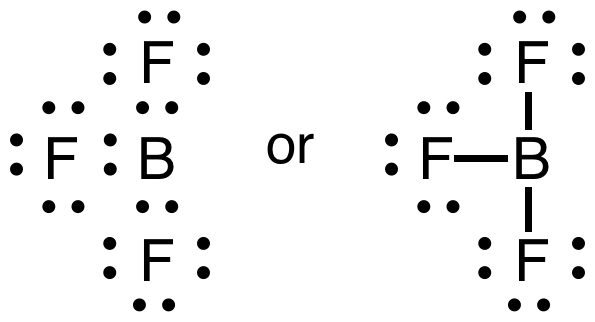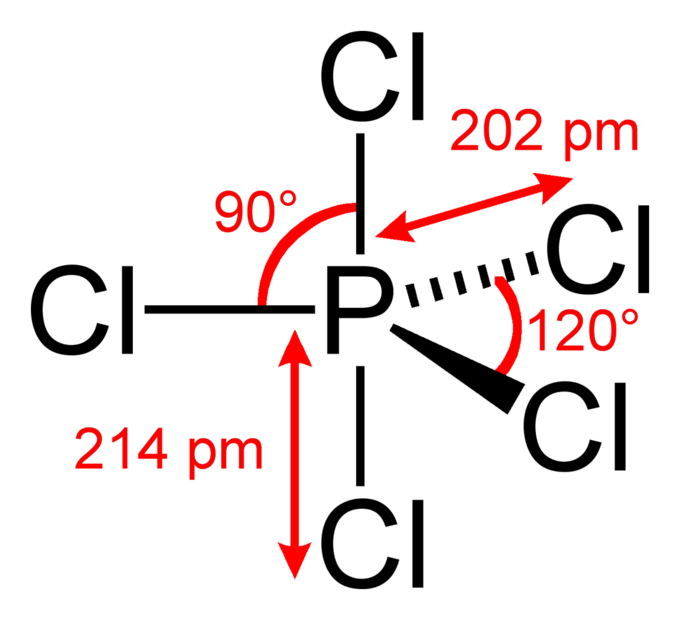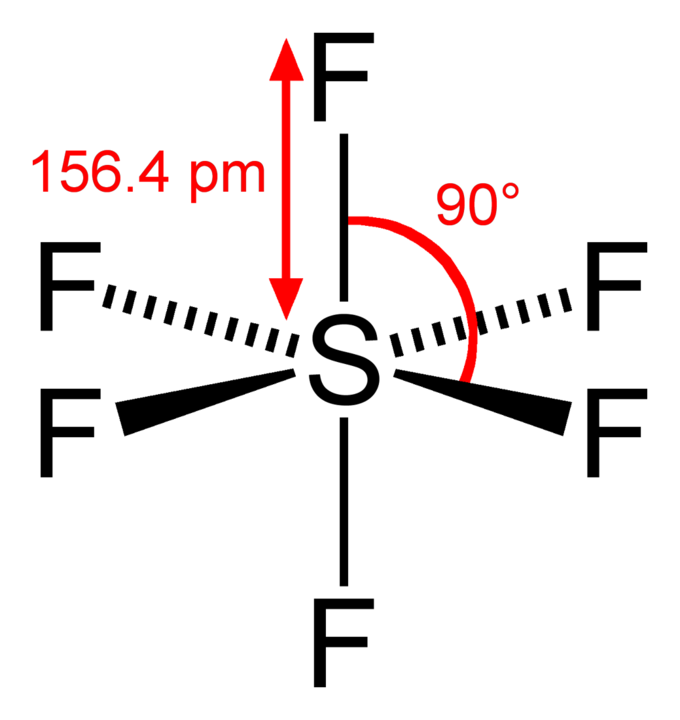The Incomplete Octet

While most elements below atomic number 20 follow the octet rule, several exceptions exist, including compounds of boron and aluminum.

Learning Objectives

Describe the ways that B, Al, Li, and H deviate from the octet rule

Key Takeaways

Key Points

• The octet rule states that atoms with an atomic number below 20 tend to combine so that they each have eight electrons in their valence shells, which gives them the same electronic configuration as a noble gas.
• The two elements that most commonly fail to complete an octet are boron and aluminum; they both readily form compounds in which they have six valence electrons, rather than the usual eight predicted by the octet rule.
• While molecules exist that contain atoms with fewer than eight valence electrons, these compounds are often reactive and can react to form species with eight valence electrons. For example, BF3 will readily bind a fluoride anion to form the BF4 anion, in which boron follows the octet rule.

Key Terms

• atomic number: The number, equal to the number of protons in an atom, that determines its chemical properties. Symbol: Z.
• valence electrons: The electrons in the outermost (valence) principal energy level of an atom that can participate in the formation of chemical bonds with other atoms.
• octet rule: Atoms gain, lose, or share electrons with other atoms in order to fill their valence level with eight electrons.

The Octet Rule and Its Exceptions

The octet rule states that atoms below atomic number 20 tend to combine so that they each have eight electrons in their valence shells, which gives them the same electronic configuration as a noble gas. The rule is applicable to the main- group elements, especially carbon, nitrogen, oxygen, and the halogens, but also to metals such as sodium and magnesium.

Valence electrons can be counted using a Lewis electron dot diagram. In carbon dioxide, for example, each oxygen shares four electrons with the central carbon. These four electrons are counted in both the carbon octet and the oxygen octet because they are shared.Carbon dioxide: A Lewis dot diagram for carbon dioxide.

Hydrogen and Lithium

However, many atoms below atomic number 20 often form compounds that do not follow the octet rule. For example, with the duet rule of the first principal energy level, the noble gas helium, He, has two electrons in its outer level. Since there is no 1p subshell, 1s is followed immediately by 2s, and thus level 1 can only have at most two valence electrons. Hydrogen only needs one additional electron to attain this stable configuration, through either covalent sharing of electrons or by becoming the hydride ion (:H), while lithium needs to lose one by combining ionically with other elements. This leads to hydrogen and lithium both having two electrons in their valence shell—the same electronic configuration as helium—when they form molecules by bonding to other elements.

Boron and Aluminum

There are also a variety of molecules in which there are too few electrons to provide an octet for every atom. Boron and aluminum, from Group III (or 13), display different bonding behavior than previously discussed. These atoms each have three valence electrons, so we would predict that these atoms want to bond covalently in order to gain 5 electrons (through sharing) to fulfill the octet rule. However, compounds in which boron or aluminum atoms form five bonds are never observed, so we must conclude that simple predictions based on the octet rule are not reliable for Group III.

Consider boron trifluoride (BF3). The bonding is relatively simple to model with a Lewis structure if we allow each valence level electron in the boron atom to be shared in a covalent bond with each fluorine atom. In this compound, the boron atom only has six valence shell electrons, but the octet rule is satisfied by the fluorine atoms.Lewis structure of boron trifluoride: Each pair of dots represents a pair of electrons. When placed between two atoms, the electrons are in a bond. A bond can be drawn as a line between two atoms, which also indicates two electrons. Notice that the central boron atom has only 6 electrons in the final Lewis diagram/structure of this molecule.

We might conclude from this one example that boron atoms obey a sextet rule. However, boron will form a stable ion with hydrogen, BH4, in which the boron atom does have a complete octet. In addition, BF3 will react with ammonia (NH3), to form a stable compound, NH3BF3, for which a Lewis structure can be drawn that shows boron with a complete octet.Boron trifluoride-ammonia complex: This covalent compound (NH3BF3) shows that boron can have an octet of electrons in its valence level.

Compounds of aluminum follow similar trends. Aluminum trichloride (AlCl3), aluminum hydride (AlH3), and aluminum hydroxide (Al(OH)3) indicate a valence of three for aluminum, with six valence electrons in the bonded molecule. However, the stability of aluminum hydride ions (AlH4) indicates that Al can also support an octet of valence shell electrons.

Although the octet rule can still be of some utility in understanding the chemistry of boron and aluminum, the compounds of these elements are harder to predict than for other elements.

Odd-Electron Molecules

Molecules with an odd number of electrons disobey the octet rule.

Learning Objectives

Describe the deviation from the octet rule by free radicals

Key Takeaways

Key Points

• While the majority of compounds formed from atoms below atomic number 20 follow the octet rule, there are many examples of compounds that do not.
• Having an odd number of electrons in a molecule guarantees that it does not follow the octet rule, because the rule requires eight electrons (or two for hydrogen) around each atom.
• The most commonly encountered stable species that exist with an odd number of electrons are nitrogen oxides, such as nitric oxide (NO) and nitrogen dioxide (NO2), both of which are free radicals and disobey the octet rule.

Key Terms

• metastable: Of or pertaining to a physical or chemical state that is relatively long-lived, but may decay to a lower energy state when perturbed.
• free radical: Any molecule, ion, or atom with one or more unpaired electrons. They vary in reactivity and stability from highly reactive, occurring as transient (short-lived) species, to metastable.
• octet rule: Atoms lose, gain, or share electrons in order to have a full valence shell of eight electrons. Hydrogen is an exception because it can hold a maximum of two electrons in its valence level.

Some elements, most notably nitrogen, can form compounds that do not obey the octet rule. One class of such compounds are those that have an odd number of electrons. As the octet rule requires eight electrons around each atom, a molecule with an odd number of electrons must disobey the octet rule. Molecules with unpaired electrons are termed ‘free radicals.’ While typically highly unstable, and therefore highly reactive, some free radicals exhibit stability of days, months, or even years. These latter compounds are said to be ‘ metastable,’ meaning they will decompose or react if given enough time, but are stable enough for a considerable amount of time, from days to even years, when subjected to only minor disturbances.

Recall that the Lewis structure of a molecule must depict the total number of  valence electrons from all the atoms which are bonded together.

Nitric oxide has the formula NO. The total number of valence electrons is 5+6=11. Therefore, no matter how electrons are shared between the nitrogen and oxygen atoms, there is no way for nitrogen to have an octet. It will have seven electrons, assuming that the oxygen atom does satisfy the octet.Nitric oxide: Nitric oxide (NO) is an example of a stable free radical. It does not obey the octet rule on the nitrogen atom. Each line around the atoms represents a pair of electrons.

Nitric oxide is a by-product of combustion reactions that occur in engines, like those in automobile engines and fossil fuel power plants. It is also produced naturally during the electrical discharge of lightning during thunderstorms.

Nitrogen dioxide is the chemical compound with the formula NO2. Again, nitrogen dioxide does not follow the octet rule for one of its atoms, namely nitrogen. The total number of valence electrons is 5+2(6)=17. There is persistent radical character on nitrogen because it has an unpaired electron. The two oxygen atoms in this molecule follow the octet rule.Nitrogen dioxide: Nitrogen dioxide is another stable molecule that disobeys the octet rule. Note the seven electrons around nitrogen. Formal charges and the molecule’s resonance structures are indicated.

Nitrogen dioxide is an intermediate in the industrial synthesis of nitric acid, millions of tons of which is produced each year. This reddish-brown toxic gas has a characteristic sharp, biting odor and is a prominent air pollutant.

The Expanded Octet

Main group elements in the third period and below form compounds that deviate from the octet rule by having more than 8 valence electrons.

Learning Objectives

Explain why some elements can form an expanded octet

Key Takeaways

Key Points

• Main group elements that form more bonds than would be predicted by the octet rule are called hypervalent compounds, and have what is known as an ‘ expanded octet,’ meaning that there are more than eight electrons around one atom.
• The octet rule can be ‘expanded’ by some elements by utilizing the d- orbitals found in the third principal energy level and beyond. Sulfur, phosphorus, silicon, and chlorine are common examples of elements that form an expanded octet.
• Phosphorus pentachloride (PCl5) and sulfur hexafluoride (SF6) are examples of molecules that deviate from the octet rule by having more than 8 electrons around the central atom.

Key Terms

• main group element: Elements that are not part of the transition metal block in the periodic table.
• expanded octet: A case where an atom shares more than eight electrons with its bonding partners.
• hypervalent molecule: A molecule that contains an atom from a main group element which deviates from the octet rule by sharing more than eight electrons.

Deviations from the Octet Rule

A hypervalent molecule is a molecule that contains one or more main group elements that bear more than eight electrons in their valence levels as a result of bonding. Phosphorus pentachloride (PCl5), sulfur hexafluoride (SF6), chlorine trifluoride (ClF3), and the triiodide ion (I3) are examples of hypervalent molecules.

For the elements in the second period of the periodic table (principal energy level n=2), the s2p6 electrons comprise the octet, and no d sublevel exists. As a result, the second period elements (more specifically, the nonmetals C, N, O, F) obey the octet rule without exceptions.Phosphorus pentachloride: In the PCl5 molecule, the central phosphorus atom is bonded to five Cl atoms, thus having 10 bonding electrons and violating the octet rule. The overall geometry of the molecule is depicted (trigonal bipyramidal), and bond angles and lengths are highlighted.

However, some of the third-period elements (Si, P, S, and Cl) have been observed to bond to more than four other atoms, and thus need to involve more than the four pairs of electrons available in an s2p6 octet. This is possible because for n=3, the d sublevel exists, and it has five d orbitals. Although the energy of empty 3d-orbitals is ordinarily higher than that of the 4s orbital, that difference is small and the additional d orbitals can accommodate more electrons. Therefore, the d orbitals participate in bonding with other atoms and an expanded octet is produced. Examples of molecules in which a third period central atom contains an expanded octet are the phosphorus pentahalides and sulfur hexafluoride.Sulfur hexafluoride: In the SF6 molecule, the central sulfur atom is bonded to six fluorine atoms, so sulfur has 12 bonding electrons around it. The overall geometry of the molecule is depicted (tetragonal bipyramidal, or octahedral), and bond angles and lengths are highlighted.

For atoms in the fourth period and beyond, higher d orbitals can be used to accommodate additional shared pairs beyond the octet. The relative energies of the different kinds of atomic orbital reveal that energy gaps become smaller as the principal energy level quantum number (n) increases, and the energetic cost of using these higher orbitals to accommodate bonding electrons becomes smaller.